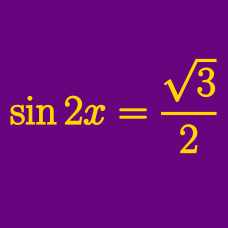Geometry

# Trigonometric Equations - Triple Angle Formula

In the domain $[0, 2\pi]$, how many solutions are there to

$\cos 3 \theta = 4 \cos \theta + 3 ?$

If provided that $\sin x+\cos x=\frac{1}{6},$ what is the value of $\sin 3x-\cos 3x ?$

Suppose that $\displaystyle \cos \theta=\frac{\sqrt{2}}{4}$ for $\displaystyle 0 < \theta < \frac{\pi}{2}.$ Then $\sin 3\theta+\cos 3\theta$ can be expressed as $\sin 3\theta+\cos 3\theta=\frac{a\sqrt{2}+b\sqrt{14}}{8}$ for rational numbers $a$ and $b.$ What is the value of $3a+8b ?$

If provided that $\sin x+\cos x=\frac{\sqrt{3}}{5},$ where $\sin x > \cos x,$ what is the value of $\sin 3x+\cos 3x ?$

Suppose that $\sin 3\theta=\sin 2\theta$ for $\displaystyle 0 < \theta < \frac{\pi}{2}.$ Then what is the value of $20\cos^2 \theta-10\cos \theta ?$

×